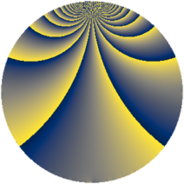# Properties

 Label 169.2.iLevel $169$ Weight $2$ Character orbit 169.i Rep. character $\chi_{169}(3,\cdot)$ Character field $\Q(\zeta_{39})$ Dimension $336$ Newform subspaces $1$ Sturm bound $30$ Trace bound $0$

# Related objects

## Defining parameters

 Level: $$N$$ $$=$$ $$169 = 13^{2}$$ Weight: $$k$$ $$=$$ $$2$$ Character orbit: $$[\chi]$$ $$=$$ 169.i (of order $$39$$ and degree $$24$$) Character conductor: $$\operatorname{cond}(\chi)$$ $$=$$ $$169$$ Character field: $$\Q(\zeta_{39})$$ Newform subspaces: $$1$$ Sturm bound: $$30$$ Trace bound: $$0$$

## Dimensions

The following table gives the dimensions of various subspaces of $$M_{2}(169, [\chi])$$.

Total New Old
Modular forms 384 384 0
Cusp forms 336 336 0
Eisenstein series 48 48 0

## Trace form

 $$336q - 26q^{2} - 26q^{3} - 12q^{4} - 26q^{5} - 26q^{6} - 26q^{7} - 26q^{8} - 12q^{9} + O(q^{10})$$ $$336q - 26q^{2} - 26q^{3} - 12q^{4} - 26q^{5} - 26q^{6} - 26q^{7} - 26q^{8} - 12q^{9} - 22q^{10} - 26q^{11} - 34q^{12} - 13q^{13} - 30q^{14} + 26q^{15} - 8q^{16} - 24q^{17} - 104q^{18} - 13q^{19} - 26q^{20} - 26q^{21} + 19q^{22} + 67q^{23} + 52q^{24} - 58q^{25} - 26q^{26} - 38q^{27} - 26q^{28} - 22q^{29} - 120q^{30} + 26q^{31} + 117q^{32} - 26q^{33} + 39q^{34} - 18q^{35} - 2q^{36} - 26q^{37} + 41q^{38} + 26q^{39} + 67q^{40} - 26q^{41} - 270q^{42} - 16q^{43} + 39q^{45} - 39q^{47} + 31q^{48} + 72q^{49} - 26q^{50} + 19q^{51} + 39q^{52} + 36q^{53} - 182q^{54} + 116q^{55} - 10q^{56} + 52q^{57} + 26q^{58} - 234q^{59} + 78q^{60} - 16q^{61} + 53q^{62} + 39q^{63} - 82q^{64} - 26q^{65} - 135q^{66} + 130q^{67} + 51q^{68} + 25q^{69} + 156q^{70} - 104q^{71} + 143q^{72} - 26q^{73} + 79q^{74} + 152q^{75} - 104q^{76} - 58q^{77} + 104q^{78} - 46q^{79} - 13q^{80} + 4q^{81} + 118q^{82} - 286q^{83} + 221q^{84} + 130q^{85} + 52q^{86} + 90q^{87} - 204q^{88} + 117q^{89} - 86q^{90} + 26q^{91} - 22q^{92} + 39q^{93} + 126q^{94} - 47q^{95} + 143q^{96} + 52q^{97} - 26q^{98} - 52q^{99} + O(q^{100})$$

## Decomposition of $$S_{2}^{\mathrm{new}}(169, [\chi])$$ into newform subspaces

Label Dim. $$A$$ Field CM Traces $q$-expansion
$$a_2$$ $$a_3$$ $$a_5$$ $$a_7$$
169.2.i.a $$336$$ $$1.349$$ None $$-26$$ $$-26$$ $$-26$$ $$-26$$